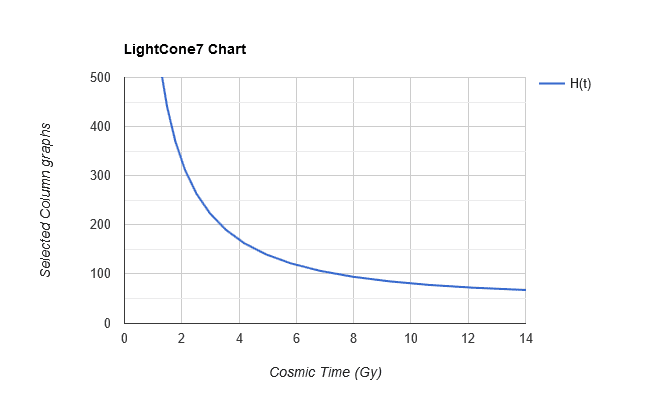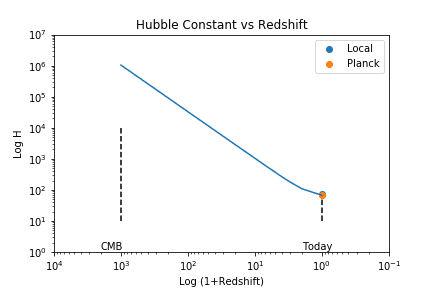# Gaia parallaxes might reduce tension for Hubble constant

• I
• mfb
• Featured
The Hubble constant is the rate of expansion now. It isn't a fundamental constant like the speed of light: it's just the value of the ##H(t)## function where ##t## is set to now.f

#### mfb

Mentor
In the last years a discrepancy between two methods to measure the Hubble constant appeared. Measurements based on redshift and the cosmic distance ladder produced results of about 73 km/(s*Mpc) while measurements based on the cosmic microwave background lead to results of about 68 km/(s*Mpc). While not completely outside the uncertainties the difference was still curious.

There are two additional independent measurements of the Hubble constant but so far they don't have the necessary precision to help: Gravitational waves from binary neutron star mergers (##70^{+12}_{-8}## from LIGO/Virgo based one one event) and multiply imaged quasars (##72.5 \pm 2.2## from H0LiCOW).

Luckily we have Gaia. More precise parallax measurements show distances could have been underestimated. A larger distance for the same redshift means a smaller Hubble constant, closer to the value from CMB measurements. Preprint:
GAIA Cepheid parallaxes and 'Local Hole' relieve H0 tension

•TEFLing, QuantumQuest, bapowell and 1 other person
I saw this. Very interesting. I wonder what Riess, et.al. have to say about it. Could the Cepheid distances really be that far off (~7%)?

•TEFLing
I saw this. Very interesting. I wonder what Riess, et.al. have to say about it. Could the Cepheid distances really be that far off (~7%)?
Easily. Cepheids are a very dirty distance measurement, and their calibration involves measurement of a relatively small number of stars which have alternative distance measurements. If those stars that can be measured in independent ways are unusual in any respect, it can throw off the measurement by quite a lot.

•TEFLing
As expected, Riess, et.al. have published a rebuttal:

https://arxiv.org/pdf/1810.03526.pdf
Very interesting! I'm glad they responded. Adam Riess is a great data scientist, and I generally trust his conclusions.

Edit:
To summarize for anybody reading this, Riess and his collaborators pointed out some serious flaws in the paper in the original post. The main ones are that they used cepheids whose distances are calibrated using an old distance measure (main sequence fitting), as well as some very bright ones that saturate the detectors (which mucks up their ability to determine the precise position of the source in an image: multiple pixels near the "true" position are maxed out and show the same color).

Once you exclude the data that shouldn't have been included, the results are consistent with other nearby measurements of ##H_0##.

They point out a few other issues, but for me this is the main take-away.

•TEFLing and bapowell
Isn't it possible that the Hubble constant has changed over time? Wouldn't these two different means of measuring the Hubble constant be essentially measuring the constant at different times in the past? Therefore, isn't it possible for them to both actually be correct?

•TEFLing
Isn't it possible that the Hubble constant has changed over time? Wouldn't these two different means of measuring the Hubble constant be essentially measuring the constant at different times in the past? Therefore, isn't it possible for them to both actually be correct?

No. The Hubble constant certainly changes with time. But both measurements are reporting the Hubble constant at the current time. The CMB measurement is taking the measured CMB radiation, calculating the best fit cosmology, and reporting what the Hubble constant extrapolates to at the current time. This is basically extrapolating forward in time over 13 billion years. The "local" measurement is a direct measurement of the Hubble constant at the current time. If there is a disagreement, it means either one measurement is wrong, or our cosmology model needs adjustment.

•TEFLing
@phyzguy you basically just proved my point. Suppose the Hubble constant has changed from 50 to 100 over the past 13 billion years. Then, measuring the CMB is essentially averaging that change over 13 billion years while the cosmic distance ladder would be measuring less than 13 billions to present.This would result in different values to be calculated. you are essentially integrating over a different length of time.

If the Hubble constant has indeed been constant for the past 13 Billion years, then I would expect both methods to get the same result.

@phyzguy you basically just proved my point. Suppose the Hubble constant has changed from 50 to 100 over the past 13 billion years. Then, measuring the CMB is essentially averaging that change over 13 billion years while the cosmic distance ladder would be measuring less than 13 billions to present.This would result in different values to be calculated. you are essentially integrating over a different length of time.

If the Hubble constant has indeed been constant for the past 13 Billion years, then I would expect both methods to get the same result.
I think you're fundamentally misunderstanding what the Hubble constant is. The Hubble constant is the rate of expansion now. It isn't a fundamental constant like the speed of light: it's just the value of the ##H(t)## function where ##t## is set to now.

The rate of expansion was certainly far faster in the past. At the time the CMB was emitted, it was tens of thousands of times the rate of expansion today.

The way in which the CMB is used to produce a measurement of the Hubble constant is not by measuring some expansion rate in the past and extrapolating that rate forward. Rather, it's done through a cosmological model. Basically, the CMB data is sensitive to three things:
1) The CMB is very sensitive to the amount of normal matter in the universe. This directly impacts the overall brightness of the CMB itself, as the CMB was emitted from the early-universe plasma which existed at that time.
2) It is very sensitive to the ratio of dark matter to normal matter. I won't go into detail, but there's a wave pattern set up in the CMB by the presence of dark matter which allows us to estimate this ratio very precisely.
3) Finally, the CMB photons themselves travel through the rest of the universe, and in doing so constrain the overall shape of the universe in the intervening time. These measurements indicate that the universe has near-zero spatial curvature.

When you combine the above three, and add in a model for dark energy (usually the cosmological constant), you also end up constraining the current rate of expansion.

A tension between nearby measurements of the Hubble constant versus CMB-based measurements can mean a few different things:
1) The nearby measurements are biased in some way.
2) The CMB measurements are biased in some way.
3) We just happen to be in an unusual spot in the universe.
4) There's something we're missing about the model that connects the nearby measurements with the CMB measurements (e.g. dark energy that varies in time rather than being a cosmological constant).

This paper tried to claim that (1) was probably the answer, but I think Riess et. al.'s response effectively removes their argument from consideration.

•Buzz Bloom, TEFLing, Orodruin and 1 other person
For visual reference, H(t) evolution in the concordance model, obtained from the CMBR data (PLANCK 2015):The supernova data places the current value of H(t) slightly above the curve. The discrepancy amounts to a few percent.

#### Attachments

•TEFLing
@phyzguy you basically just proved my point. Suppose the Hubble constant has changed from 50 to 100 over the past 13 billion years. Then, measuring the CMB is essentially averaging that change over 13 billion years while the cosmic distance ladder would be measuring less than 13 billions to present.This would result in different values to be calculated. you are essentially integrating over a different length of time.
If the Hubble constant has indeed been constant for the past 13 Billion years, then I would expect both methods to get the same result.

To add further to what kimbyd and Bandersnatch said, look at the attached plot. The Hubble constant has decreased by a huge amount since the CMB. We are extrapolating the measurements of the CMB to today along the line shown, based on the standard model of cosmology as kimbyd explained. The discrepancy that people are arguing about is the difference between the red dot and the blue dot.#### Attachments

Last edited:
•TEFLing and Imager
•TEFLing
The discussion is still ongoing.

First Cosmological Results using Type Ia Supernovae from the Dark Energy Survey: Measurement of the Hubble Constant (DES collaboration)
They use supernovae with an inverse distance ladder based on baryon acoustic oscillations. The value they find is similar to Planck.

If we assume all the values are properly measured and we don't have a weird statistical fluctuation this would point to something going on in the early universe that affects all measurements relying on the CMB.

Early Dark Energy Can Resolve The Hubble Tension
This is a proposed model to change the early universe.

•TEFLing and Bandersnatch
The discussion is still ongoing.

First Cosmological Results using Type Ia Supernovae from the Dark Energy Survey: Measurement of the Hubble Constant (DES collaboration)
They use supernovae with an inverse distance ladder based on baryon acoustic oscillations. The value they find is similar to Planck.

If we assume all the values are properly measured and we don't have a weird statistical fluctuation this would point to something going on in the early universe that affects all measurements relying on the CMB.

This is a very interesting paper, but I don't think I agree with your assessment. Their measurement of H0 does not "rely on the CMB". It uses only the SN1A and BAO measurements out to a red-shift z=0.85. This is a lookback time only about half way to the CMB. So it is a "local" measurement, although not as local as relying on the Cepheid distance ladder. The fact that this is based on SN1A and does not agree with using the SN1A calibrated from Cepheids to me implies some systematic offset in the Cepheid based distance ladder, as claimed in the first paper of this thread.

•TEFLing
Okay, maybe I should have said "early universe" only. Earlier than all other methods apart from the direct CMB measurements.
In addition BAO come from the same time as the CMB.

•TEFLing